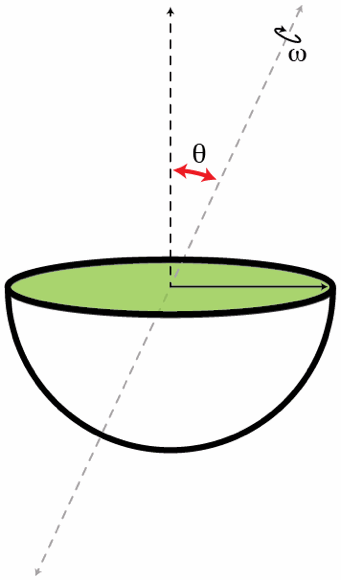# Kinetic energy of a hemisphereA solid hemisphere of radius $R=0.5~\mbox{m}$ and mass $M=1~\mbox{kg}$ rotates with angular speed $\omega=10~\text{rad/s}$ about an axis passing through its center O and making and angle $\theta=60^{\circ}$ with the vertical (see the figure below). Determine the kinetic energy in Joules of the hemisphere.

×